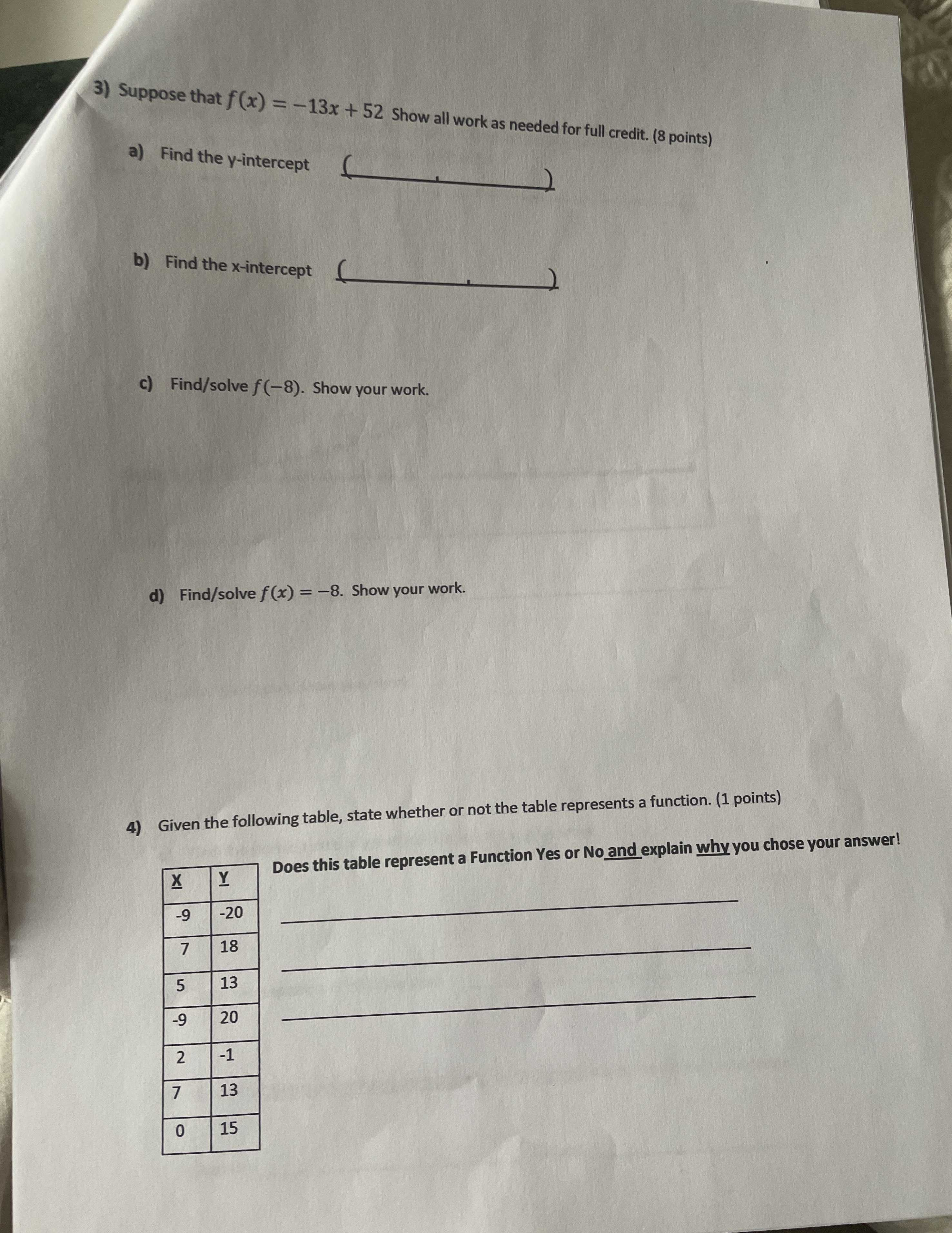### ¿Todavía tienes preguntas de matemáticas?

Pregunte a nuestros tutores expertos
Algebra
Pregunta3) Suppose that $$f ( x ) = - 13 x + 52$$ Show all work as needed for full credit.

a) Find the $$y$$ -intercept .

b) Find the $$x$$ -intercept .

c) Find/solve $$f ( - 8 )$$ . Show your work.

d) Find/solve $$f ( x ) = - 8$$ . Show your work.

4) Given the following table, state whether or not the table represents a function.

Does this table represent a Function Yes or No and explain why you chose your answer!